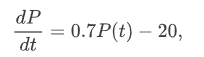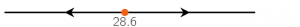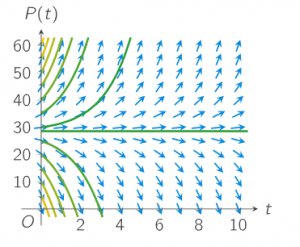# 1.6 Validation

Course subject(s) 1. Introducing Mathematical Modelling

In the previous sections you have seen the differential equationh the initial condition P(0)=30. The phase line for this differential equation isand the direction field with some possible solutions is like this:Now you will validate these solutions. Do these solutions represent realistic solutions to our problem?